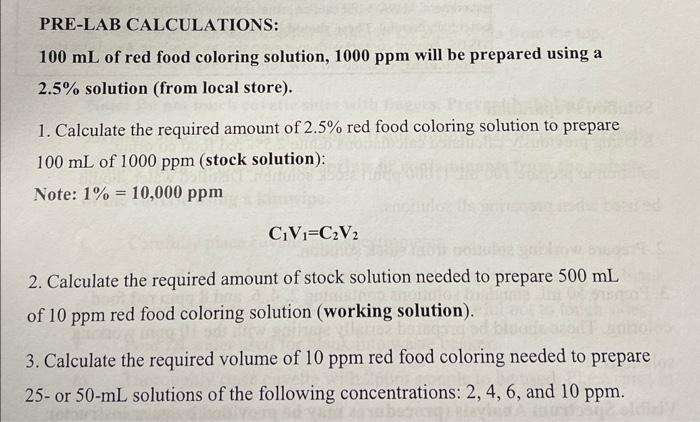Home / Expert Answers / Chemistry / pre-lab-calculations-100ml-of-red-food-coloring-solution-1000ppm-will-be-prepared-using-a-2-5-pa390

# (Solved): PRE-LAB CALCULATIONS: 100mL of red food coloring solution, 1000ppm will be prepared using a 2.5% ...PRE-LAB CALCULATIONS: of red food coloring solution, will be prepared using a solution (from local store). 1. Calculate the required amount of red food coloring solution to prepare of (stock solution): Note: 2. Calculate the required amount of stock solution needed to prepare of red food coloring solution (working solution). 3. Calculate the required volume of red food coloring needed to prepare 25 - or 50 -mL solutions of the following concentrations: , and .

We have an Answer from Expert